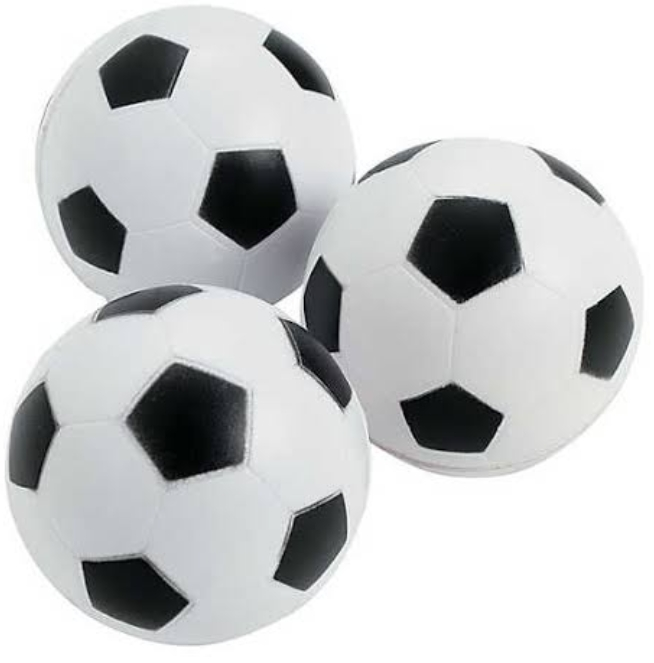# Substraction of Whole Numbers (Primary 1)

MATHEMATICS

SECOND TERM

WEEK 2

PRIMARY 1

THEME –  WHOLE NUMBERS

PREVIOUS LESSON – WRITING 1 – 5

TOPIC – SUBTRACTION

PERFORMANCE OBJECTIVES

By the end of the lesson, the pupils should have attained the following objectives (cognitive, affective and psychomotor) and should be able to –

1. Subtract from whole numbers not greater than 9.
2. subtract from whole numbers not greater than 18.
3. cross check accuracy in subtraction.

ENTRY BEHAVIOUR

INSTRUCTIONAL MATERIALS

The teacher will teach the lesson with the aid of:

1. Stones
2. Oranges
3. Bean seed
4. Balls
5. Bottle tops
6. Number beads, etc.

METHOD OF TEACHING – Choose a suitable and appropriate methods for the lessons.

Note – Irrespective of choosing methods of teaching, always introduce an activities that will arouse pupil’s interest or lead them to the lessons.

REFERENCE MATERIALS

1. Scheme of Work
2. 9 – Years Basic Education Curriculum
3. Course Book
4. All Relevant Material
5. Online Information

CONTENT OF THE LESSON

LESSON 1 AND 2

SUBTRACTION OF WHOLE NUMBERS NOT GREATER THAN 9

ACTIVITIES 1 – IDENTIFY THE OBJECTBALLS

ACTIVITIES 2 – HOW MANY BALLS

8 balls

ACTIVITIES 3 – 8  BALLS TAKE AWAY 3ACTIVITIES 3 – LET THEM READ – 8 BALLS TAKE AWAY 3 BALL

That’s, 8 minus 3ACTIVITIES 4 – COUNT THE REMAINING BALLS5 BALLS

ACTIVITIES 5 – READ 8 BALLS TAKE AWAY 3 BALLS REMANDS 5 BALL

That’s, 8 minus 3 is 5Repeat this activities on other countable objects.

LESSON 3 AND 4

SUBTRACTION OF WHOLE NUMBERS NOT GREATER THAN 18

Follow the same procedure.

PRESENTATION

• To deliver the lesson, the teacher adopts the following steps:
1. To introduce the lesson, the teacher revises the previous lesson. Based on this, he/she asks the pupils some questions;
2. Guides pupils to group a particular set of objects e.g. oranges into 1, 2, 3, 4, 5, 6,7, 8 and 9.
3. Pupil’s Activities – Group objects such as oranges, stones  etc. into 1, 2, 3, 4, 5, 6 7, 8 and 9.
4. Leads pupils to take a group of objects and takeaway a lesser number of the same objects from the group, e.g. in a group of 8oranges take away 6oranges.
5. Pupil’s Activities – Count one given group of objects and remove a lesser number of the same objects from the group.
6. Guides pupils to group a particular objects, e.g. bean seed into sets of 18, 17, 16, 15, 14, 13, 12,11, 10, 9, 8.
7. Pupil’s Activities – Group objects such as bean seed into 18, 17, 16, 15, 14, 13, 12,11, 10, 9, 8.
8. Guides pupils to count a group of objects and takeaway a lesser number of the same objects from the group, e.g. in a group of 16bean seed take away 10 bean seeds.
9. Pupil’s Activities – Count one given group of objects and remove a lesser number of the same object from the group.
10. Leads pupils to give examples of everyday life where accuracy of subtraction is required. Emphasizes the importance of accuracy of subtraction in everyday activities.
11. Pupil’s Activities – Give examples of everyday life where accuracy in subtraction is needed.

CONCLUSION

• To conclude the lesson for the week, the teacher revises the entire lesson and links it to the following week’s lesson.
• Next Lesson – Addition

LESSON EVALUATION

Pupils to:

1. subtract given whole numbers not greater than 9.
2. subtract given whole numbers not greater than18.
3. mention two examples of everyday activities where accuracy in subtraction is needed.